# 9.1 General Form of a Conic

$$\newcommand{\vecs}{\overset { \rightharpoonup} {\mathbf{#1}} }$$ $$\newcommand{\vecd}{\overset{-\!-\!\rightharpoonup}{\vphantom{a}\smash {#1}}}$$$$\newcommand{\id}{\mathrm{id}}$$ $$\newcommand{\Span}{\mathrm{span}}$$ $$\newcommand{\kernel}{\mathrm{null}\,}$$ $$\newcommand{\range}{\mathrm{range}\,}$$ $$\newcommand{\RealPart}{\mathrm{Re}}$$ $$\newcommand{\ImaginaryPart}{\mathrm{Im}}$$ $$\newcommand{\Argument}{\mathrm{Arg}}$$ $$\newcommand{\norm}{\| #1 \|}$$ $$\newcommand{\inner}{\langle #1, #2 \rangle}$$ $$\newcommand{\Span}{\mathrm{span}}$$ $$\newcommand{\id}{\mathrm{id}}$$ $$\newcommand{\Span}{\mathrm{span}}$$ $$\newcommand{\kernel}{\mathrm{null}\,}$$ $$\newcommand{\range}{\mathrm{range}\,}$$ $$\newcommand{\RealPart}{\mathrm{Re}}$$ $$\newcommand{\ImaginaryPart}{\mathrm{Im}}$$ $$\newcommand{\Argument}{\mathrm{Arg}}$$ $$\newcommand{\norm}{\| #1 \|}$$ $$\newcommand{\inner}{\langle #1, #2 \rangle}$$ $$\newcommand{\Span}{\mathrm{span}}$$$$\newcommand{\AA}{\unicode[.8,0]{x212B}}$$

Conics are a family of graphs that include parabolas, circles, ellipses and hyperbolas. All of these graphs come from the same general equation and by looking and manipulating a specific equation you can learn to tell which conic it is and how it can be graphed.

What is the one essential skill that enables you to manipulate the equation of a conic in order to sketch its graph?

Introduction to Conics

The word conic comes from the word cone which is where the shapes of parabolas, circles, ellipses and hyperbolas originate. Consider two cones that open up in opposite directions and a plane that intersects it horizontally. A flat intersection would produce a perfect circle.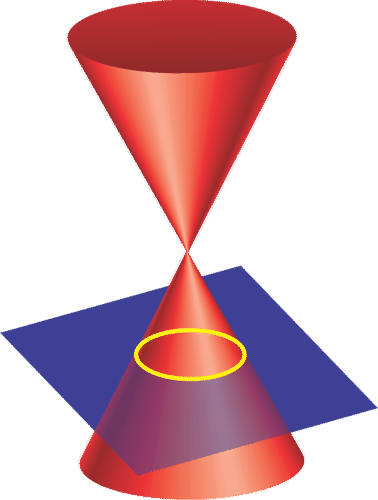To produce an ellipse, tilt the plane so that the circle becomes elongated and oval shaped. Notice that the angle that the plane is tilted is still less steep than the slope of the side of the cone.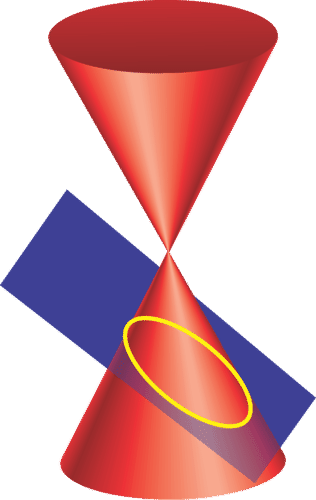As you tilt the plane even further and the slope of the plane equals the slope of the cone edge you produce a parabola. Since the slopes are equal, a parabola only intersects one of the cones.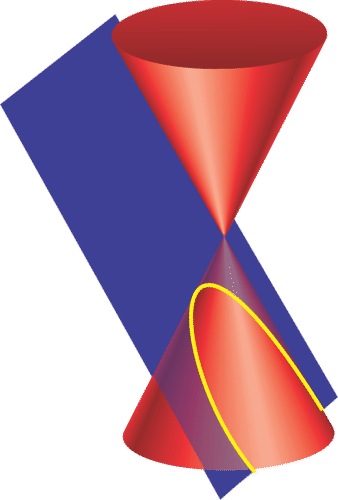Lastly, if you make the plane steeper still, the plane ends up intersecting both the lower cone and the upper cone creating the two parts of a hyperbola.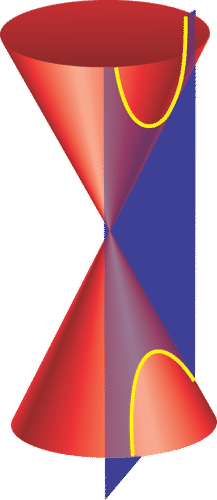The intersection of three dimensional objects in three dimensional space to produce two dimensional graphs is quite challenging. In practice, the knowledge of where conics come from is not widely used. It will be more important for you to be able to manipulate an equation into standard form and graph it in a regular coordinate plane. The regular form of a conic is:

$$A x^{2}+B x y+C y^{2}+D x+E y+F=0$$

Before you start manipulating the general form of a conic equation you should be able to recognize whether it is a circle, ellipse, parabola or hyperbola. In standard form, the two coefficients to examine are $$A$$ and $$C$$.

• For circles, the coefficients of $$x^{2}$$ and $$y^{2}$$ are the same sign and the same value: $$A=C$$
• For ellipses, the coefficients of $$x^{2}$$ and $$y^{2}$$ are the same sign and different values:
• $$A, C>0, A \neq C$$
• For hyperbolas, the coefficients of $$x^{2}$$ and $$y^{2}$$ are opposite signs: $$C<0<A$$ or $$A<0<C$$
• For parabolas, either the coefficient of $$x^{2}$$ or $$y^{2}$$ must be zero: $$A=0$$ or $$C=0$$

Each specific type of conic has its own graphing form, but in all cases the technique of completing the square is essential.

For review, let's complete the square in the expression $$x^{2}+6 x$$. and demonstrate graphically what completing the square represents.

Algebraically, completing the square just requires you to divide the coefficient of $$x$$ by 2 and square the result. In this case $$\left(\frac{6}{2}\right)^{2}=3^{2}=9$$. Since you cannot add nine to an expression without changing its value, you must simultaneously add nine and subtract nine so the net change will be zero.

$$x^{2}+6 x+9-9$$

Now you can factor by recognizing a perfect square.

$$(x+3)^{2}-9$$

Graphically the original expression $$x^{2}+6 x$$ can be represented by the area of a rectangle with sides $$x$$ and $$(x+6)$$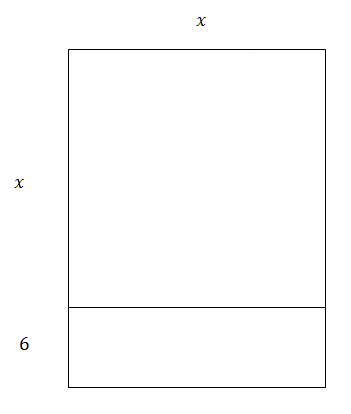The term "complete the square" has visual meaning as well algebraic meaning. The rectangle can be rearranged to be more square-like so that instead of small rectangle of area $$6 x$$ at the bottom, there is a rectangle of area $$3 x$$ on two sides of the $$x^{2}$$ square.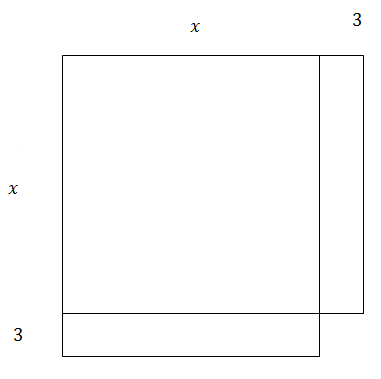Notice what is missing to make this shape a perfect square? A little corner square of 9 is missing which is why the 9 should be added to make the perfect square of $$(x+3)(x+3)$$.

## Examples

##### Example 1

Earlier, you were asked what skills you need for conics. The one essential skill that you need for conics is completing the square. If you can complete the square with two variables then you will be able to graph every type of conic.

##### Example 2

What type of conic is each of the following relations?

1. $$5 y^{2}-2 x^{2}=-25$$

a. Parabola (sideways) because the $$x^{2}$$ term is missing.

3. $$4 x^{2}+6 y^{2}=36$$

a. Ellipse because the $$x^{2}$$ and $$y^{2}$$ coefficients are different values but the same sign.

4. $$x^{2}-\frac{1}{4} y=1$$

a. Parabola (upright) because the $$y^{2}$$ term is missing.

5. $$-\frac{x^{2}}{8}+\frac{y^{2}}{4}=1$$

a. Hyperbola because the $$x^{2}$$ and $$y^{2}$$ coefficients are different signs.

6. $$-x^{2}+99 y^{2}=12$$

a. Hyperbola because the $$x^{2}$$ and $$y^{2}$$ coefficients are different signs.

##### Example 3

Complete the square for both the $$x$$ and $$y$$ terms in the following equation.

$$x^{2}+6 x+2 y^{2}+16 y=0$$

First write out the equation with space so that there is room for the terms to be added to both sides. Since this is an equation, it is appropriate to add the values to both sides instead of adding and subtracting the same value simultaneously. As you rewrite with spaces, factor out any coefficient of the $$x^{2}$$ or $$y^{2}$$ terms since your algorithm for completing the square only works when this coefficient is one.

$$x^{2}+6 x+\ldots+2\left(y^{2}+8 y+\ldots\right)=0$$

Next complete the square by adding a nine and what looks like a 16 on the left (it is actually a 32 since it is inside the parentheses).

$$x^{2}+6 x+9+2\left(y^{2}+8 y+16\right)=9+32$$

Factor

$$(x+3)^{2}+2(y+4)^{2}=41$$

##### Example 4

Identify the type of conic in each of the following relations.

1. $$3 x^{2}=3 y^{2}+18$$

a. The relation is a hyperbola because when you move the $$3 y^{2}$$ to the left hand side of the equation, it becomes negative and then the coefficients of $$x^{2}$$ and $$y^{2}$$ have opposite signs.

2. $$y=4(x-3)^{2}+2$$

a. Parabola

3. $$x^{2}+y^{2}=4$$

a. Circle

4. $$y^{2}+2 y+x^{2}-6 x=12$$

a. Circle

5. $$\frac{x^{2}}{6}+\frac{y^{2}}{12}=1$$

a. Ellipse

6. $$x^{2}-y^{2}+4=0$$

a. Hyperbola

##### Example 5

Complete the square for both $$x$$ and $$y$$ in the following equation.

$$-3 x^{2}-24 x+4 y^{2}-32 y=8$$

$$-3 x^{2}-24 x+4 y^{2}-32 y=8$$

$$-3\left(x^{2}+8 x+\underline{}\underline{}\right)+4\left(y^{2}-8 y+\underline{}\underline{}\right)=8$$

$$-3\left(x^{2}+8 x+16\right)+4\left(y^{2}-8 y+16\right)=8-48+64$$

$$-3(x+4)^{2}+4(y-4)^{2}=24$$

##### Review

Identify the type of conic in each of the following relations.

1. $$3 x^{2}+4 y^{2}=12$$

2. $$x^{2}+y^{2}=9$$

3. $$\frac{x^{2}}{4}+\frac{y^{2}}{9}=1$$

4. $$y^{2}+x=11$$

5. $$x^{2}+2 x-y^{2}+6 y=15$$

6. $$x^{2}=y-1$$

Complete the square for $$x$$ and/or $$y$$ in each of the following expressions

7. $$x^{2}+4 x$$

8. $$y^{2}-8 y$$

9. $$3 x^{2}+6 x+4$$

10. $$3 y^{2}+9 y+15$$

11. $$2 x^{2}-12 x+1$$

Complete the square for $$x$$ and/or $$y$$ in each of the following equations.

12. $$4 x^{2}-16 x+y^{2}+2 y=-1$$

13. $$9 x^{2}-54 x+y^{2}-2 y=-81$$

14. $$3 x^{2}-6 x-4 y^{2}=9$$

15. $$y=x^{2}+4 x+1$$

This page titled 9.1 General Form of a Conic is shared under a CK-12 license and was authored, remixed, and/or curated by CK-12 Foundation via source content that was edited to the style and standards of the LibreTexts platform; a detailed edit history is available upon request.